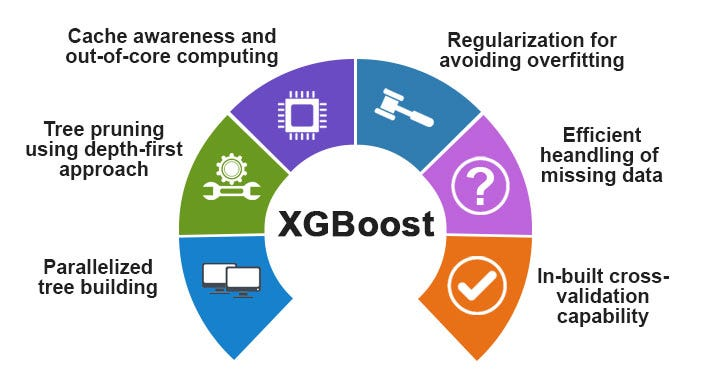The recent QUEEN of AI Algos: XGBoost, and it’s future

Source: Deep Learning on MediumUnderstanding XGBoost

In order to understand XGBoost, we must first understand Gradient Descent and Gradient Boosting.

A cost function measures how close the predicted values are, to the corresponding actual values. Ideally, we want as little difference as possible between the predicted values and the actual values. Thus, we want the cost function to be minimized.

The weights associated with a trained model, cause it to predict values that are close to the actual values. Thus, the better the weights associated with the model, the more accurate are the predicted values and the lower is the cost function. With more records in the training set, the weights are learned and then updated.

Gradient Descent is an iterative optimization algorithm. It is a method to minimize a function having several variables. Thus, Gradient Descent can be used to minimize the cost function. It first runs the model with initial weights, then seeks to minimize the cost function by updating the weights over several iterations.